Let's learn the correct Pyraminx notation

Okay, so you want to learn the correct notations for the Pyraminx. The Pyraminx is an extremely easy to puzzle to solve and doesn't use the same complex notations as larger puzzles.

In the images below the part of the puzzle that is greyed out is the part of the puzzle which does not move. The pieces in colour are the pieces that we will rotate.

Standard Notation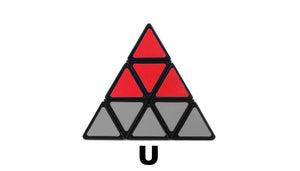When performing Pyraminx algorithms an UPPERCASE 'U' means you turn the top layer including the tip in a clockwise or anti-clockwise rotation.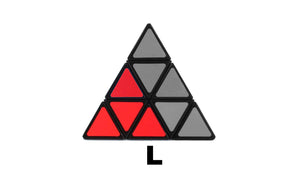When performing Pyraminx algorithms an UPPERCASE 'L' means you turn the left-hand layer including the tip in a clockwise or anti-clockwise rotation.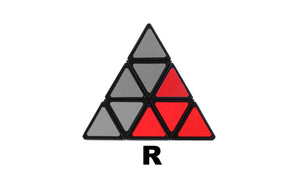When performing Pyraminx algorithms an UPPERCASE 'R' means you turn the right-hand layer including the tip in a clockwise or anti-clockwise rotation.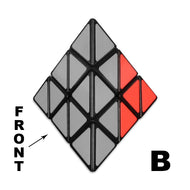When performing Pyraminx algorithms an UPPERCASE 'B' means you turn the back most layer including the tip in a clockwise or anti-clockwise rotation.

Notation for the Tips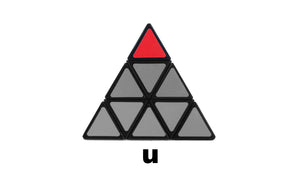When performing Pyraminx algorithms a LOWERCASE 'u' means you turn the up most tip in a clockwise or anti-clockwise rotation.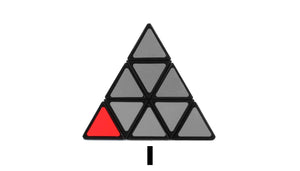When performing Pyraminx algorithms a LOWERCASE 'l' means you turn the far left-hand tip in a clockwise or anti-clockwise rotation.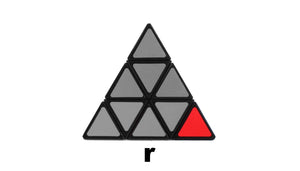When performing Pyraminx algorithms a LOWERCASE 'r' means you turn the far right-hand tip in a clockwise or anti-clockwise rotation.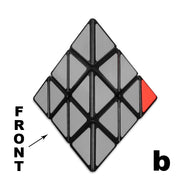When performing Pyraminx algorithms an LOWERCASE 'b' means you turn the back most tip in a clockwise or anti-clockwise rotation.